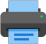Lista de exercícios do ensino médio para impressãoSelect all.
print selected exercises
The numbers $\phantom{X}\sqrt[\Large 4]{5}\;$, $\phantom{X}\sqrt[\Large 3]{3}\phantom{X}$ and $\phantom{X}\sqrt{2}\;\;$ are arranged:
a)
in descending order
b)
in ascending order
c)
in non-descending order
d)
the value of the last term is half of the sum of the first term and the second term
e)
none of these